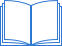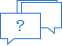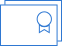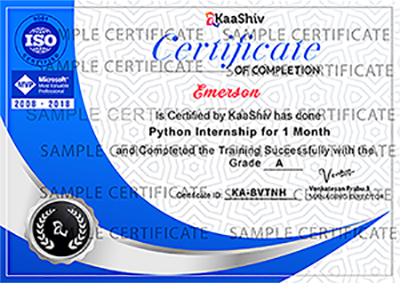# MATLAB

5 (1,225 ratings)

MATLAB is a programming language developed by MathWorks. It started out as a matrix programming language where linear algebra programming was simple. It can be run both under interactive sessions and as a batch job. This internship gives you aggressively a gentle introduction of MATLAB programming language. It is designed to give students fluency in MATLAB programming language. Problem-based MATLAB examples have been given in simple and easy way to make your learning fast and effective.

This internship has been prepared for the beginners to help them understand basic to advanced functionality of MATLAB. After completing this internship you will find yourself at a moderate level of expertise in using MATLAB from where you can take yourself to next levels.

We assume you have a little knowledge of any computer programming and understand concepts like variables, constants, expression, statements, etc. If you have done programming in any other high-level programming language like C, C++ or Java, then it will be very much beneficial and learning MATLAB will be like a fun for you.

Syllabus
5 Days
10 Days
15 Days
20 Days
1 Month
2 Months / 6 Weeks
3 Months
How it worksCoursework
Each course is like an interactive textbook, featuring pre-recorded videos, quizzes and projects.